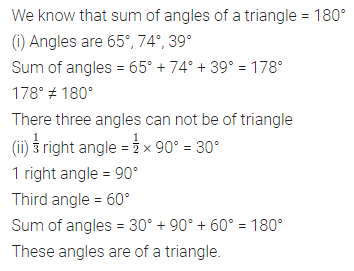# ML Aggarwal Class 7 Solutions for ICSE Maths Chapter 11 Triangles and its Properties Ex 11.2

## ML Aggarwal Class 7 Solutions for ICSE Maths Chapter 11 Triangles and its Properties Ex 11.2

Question 1.
Find the value of the unknown exterior angle x in each of the following diagrams:Solution: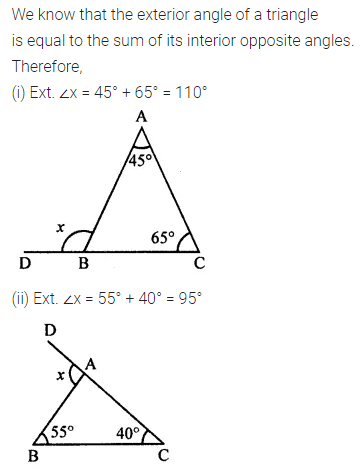Question 2.
Find the value of the unknown interior angle x in each of the following diagrams: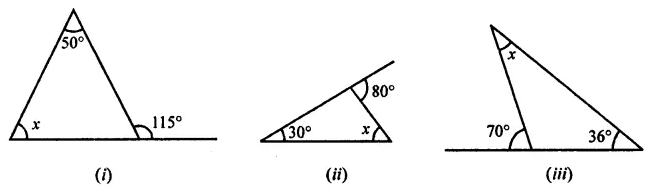Solution: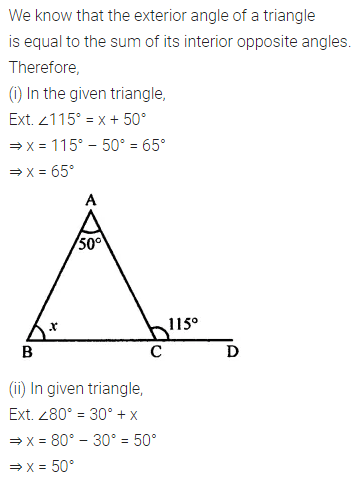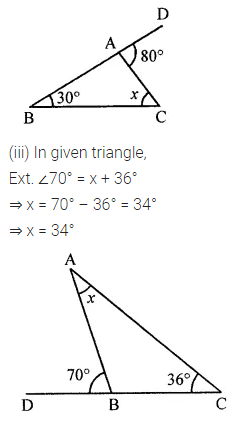Question 3.
Find the value of x in each of the following diagrams: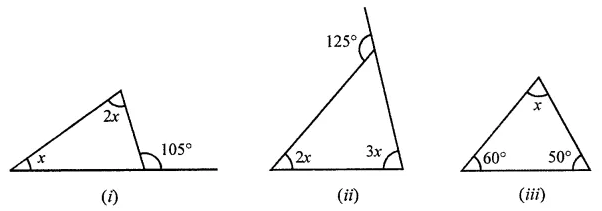Solution: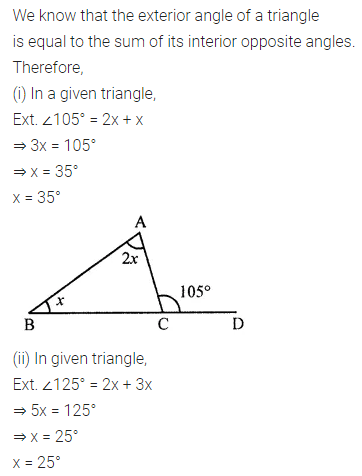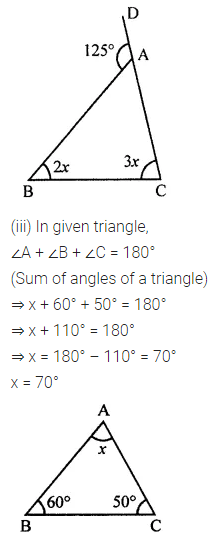Question 4.
Find the value of unknown x in each of the following:Solution:Question 5.
Find the values of x and y in each of the following diagrams:Solution: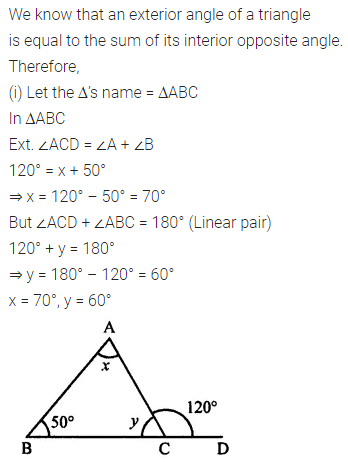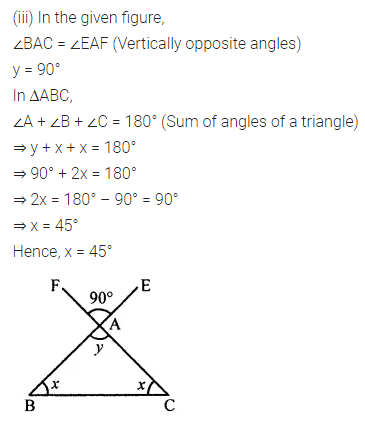Question 6.
Find the values of x and y in each of the following diagrams: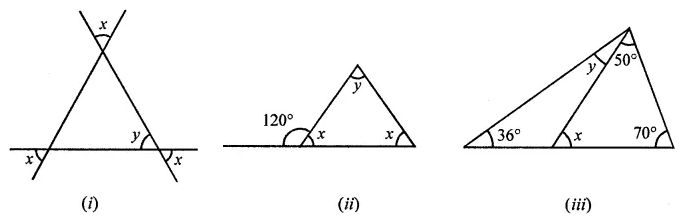Solution: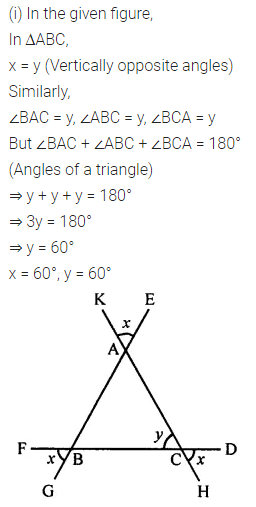Question 7.
In the adjoining figure, find the size of each lettered angle.Solution: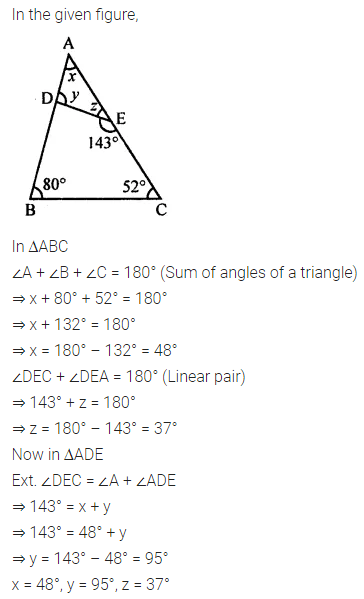Question 8.
One of the angles of a triangle measures 80° and the other two angles are equal. Find the measure of each of the equal angles.
Solution:Question 9.
If one angle of a triangle is 60° and the other two angles are in the ratio 2 : 3, find these angles.
Solution:Question 10.
If the angles of a triangle are in the ratio 1 : 2 : 3, find the angles. Classify the triangle in two different ways.
Solution: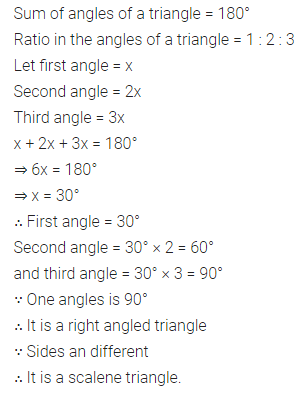Question 11.
Can a triangle have three angles whose measures are
(i) 65°, 74°, 39°?
(ii) $$\frac { 1 }{ 3 }$$ right angle, 1 right angle, 60°?
Solution: